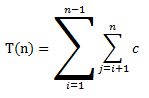# Implementation of Selection Sort with C# and analysis of running time on best, worst and average case

 Category: Data Structure And Algorithms Tags:Selection Sort Code Files

## Introduction

Previously we analyzed the insertion sort which runs on O(n2) average running time and works as we sort deck of card while playing. Selection sort is similar to insertion sort but here we do not look for the correct position of an element instead we traverse the array and find smallest element in each iteration and insert them to lower indexes and we again do the same until we are finished re-arranging whole array.

## Implementation

So the code is given below in c#

```public class SelectionSort
{
public int[] A;
public SelectionSort(int[] array)
{
A=array;
}
public int[] Sort()
{
if(A.Length > 0)
{
int min, index;
for(int i=0;i<A.Length-1;i++)
{
min = A[i];
index = i;
for(int j=i+1;j<A.Length;j++)
{
if(A[j] < min)
{
min = A[j];
index = j;
}
}
A[index] = A[i];
A[i] = min;
}
}          return A;
}
}```

In above code we have outer loop runs from first element to second last element(i<A.Length-1) and inner loop runs from second element to last, first concentrate on inner loop which is finding the smallest element in the array and replace it to lowest index, we first find the lowest element in array and place it to first position then we look for second lowest element in array and place it to second position and so on. outer loop runs till second last element only because last element will obviously on its final position. Let's run above code:

`SelectionSort selectionSort = new SelectionSort(new int[] { 2, 1, 7, 4, 9, 3, 6 });var sortedArr = selectionSort.Sort();foreach (int ele in sortedArr)    Console.WriteLine(ele);Console.ReadLine();`

Output

1

2

3

4

6

7

9

## Analysis

In case of Selection Sort, Worst, Average and best case running time will be same because whatever the input elements sequence the algorithm is going to traverse the whole array unlike insertion sort which doesn't traverse if array is already sorted and runs under O(n) running time in best case.=> T(n) = c [ (n-1) + (n-2) + ..... + 2 + 1 ]

=> T(n) = c [(n-1)n/2]

=> T(n) = c [ (n2 - n)/2 ]              (Quadratic equation)

=> T(n) = O(n2)

So the running time of selection sort is O(n2) which is not even better than the insertion sort.

 Like0 People
Last modified on 29 October 2018Nikhil Joshi Ceo & Founder at Dotnetlovers Atricles: 132 Questions: 9 Given Best Solutions: 9 *

## Comments:

No Comments Yet

You are not loggedin, please login or signup to add comments:

## Existing User

Login via:## New Userx